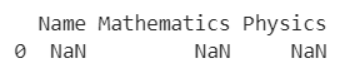# How To Create An Empty Pandas Dataframe With Only Column Names?

A DataFrame is the primary data structure of the Pandas library and is commonly used for storing and working with tabular data. A common operation that could be performed on such data is to first create an empty dataframe containing only the column names so that the information could be added later in a convenient manner.

To start working with Pandas, we first need to import it in Python code:

### Python 3 Code :

``import pandas as pd``

## Running Example

Let us understand this operation with the help of an example. Consider the following DataFrame containing students with names and their corresponding marks for two subjects, Mathematics and Physics. Initially, if we do not have the data of the students to add into the dataframe, we must construct an empty dataframe first.

On printing this empty dataframe once created, it will look as follows :

``````Empty DataFrame

Columns: [Name, Mathematics, Physics]

Index: []``````

Since the dataframe in consideration here is an empty one, the first line of the output says Empty dataframe.

The next line displays a list containing the column names (or labels) of the dataframe. The last line displays a list representing the index of the dataframe, here an empty one.

Let us look at different ways of performing this operation of creating an empty dataframe:

## Create An Empty Pandas Dataframe Using Pandas.dataframe() function with columns list

In this method, we use the Pandas.dataframe() function to create a new empty Pandas dataframe, with only column names.

A list containing the desired column labels is assigned to the columns parameter. Here, it is represented by [‘Name’, ‘Mathematics’, ‘Physics’].

Let us look at the code and corresponding output for this method.

### Python 3 Code :

``````import pandas as pd

# DataFrame containing column names only
df = pd.DataFrame(columns = ['Name', 'Mathematics', 'Physics'])

# Print the new dataframe
print(df)``````

Output :

``````Empty DataFrame

Columns: [Name, Mathematics, Physics]

Index: []``````

If you want to get the output in a more elegant manner, we pass the parameter as a list containing one element,  here. Let us look at the code and corresponding output for this method.

### Python 3 Code :

``````import pandas as pd

# DataFrame for the dictionary
df = pd.DataFrame(columns = ['Name', 'Mathematics', 'Physics'], index = )

# Print the new dataframe
print(df)``````

Output :## Create An Empty Pandas Dataframe Using Pandas.dataframe() function with data dictionary

In this method, we use the Pandas.dataframe() function to create a new empty Pandas dataframe, with only column names.

Here, data is a dictionary we created to initialize the DataFrame.

For this, we use the DataFrame() function of the Pandas library which takes the dictionary as an argument and returns the required DataFrame.

Let us look at the code and corresponding output for this method.

### Python 3 Code :

``````import pandas as pd

# Dictionary for our data
data = {'Name' : [], 'Mathematics' : [], 'Physics' : []}

# DataFrame for the dictionary
df = pd.DataFrame(data)

# Printing the DataFrame
print(df)``````

Output :

``````Empty DataFrame

Columns: [Name, Mathematics, Physics]

Index: []``````

If you want to get the output in a more elegant manner, we pass the parameter as a list containing one element,  here. Let us look at the code and corresponding output for this method.

### Python 3 Code :

``````import pandas as pd

# Dictionary for our data
data = {'Name' : [], 'Mathematics' : [], 'Physics' : [], index = }

# DataFrame for the dictionary
df = pd.DataFrame(data)

# Printing the DataFrame
print(df)``````

Output :## Conclusion

In this topic, we have learned to add an empty column to an existing DataFrame, following a running example of test scores of students in different subjects, thus giving us an intuition of how this concept could be applied in real-world situations. Feel free to reach out to info.javaexercise@gmail.com in case of any suggestions.

Trending